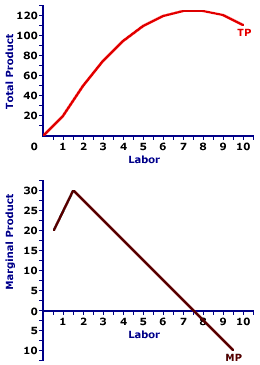Thursday  July 9, 2020
 AmosWEB means Economics with a Touch of Whimsy!ZERO BOND: Also termed a zero coupon bond, a bond that does not pay interest, in which the return is generated by the difference between the purchase price and the face value paid at maturity. Because they do not pay interest, zero bonds are sold at a discount. For example, a \$10,000 zero bond that matures in one year, would generate a 10% return if it sold at a discount of \$9,000.TOTAL PRODUCT AND MARGINAL PRODUCT:

A mathematical connection between marginal product and total product stating that marginal product IS the slope of the total product curve. If the total product curve has a positive slope (that is, is upward sloping), then marginal product is positive. If the total product curve has a negative slope (downward sloping), then marginal product is negative. If the total product curve has a zero slope (horizontal), then marginal product is zero.
The relation between total product and marginal product is one of several that reflect the general relation between a total and the corresponding marginal. There general relation is this: A marginal is the slope of the total curve. Marginal is another term for slope.

The general relation between total and marginal surfaces throughout the study of economics, especially utility (total utility and marginal utility), cost (total cost and marginal cost), revenue (total revenue and marginal revenue), and consumption (consumption expenditures and marginal propensity to consume).

### Making Tacos

Total and Marginal ProductThis two-paneled graph for the hourly production of Super Deluxe TexMex Gargantuan Tacos (with sour cream and jalapeno peppers) that visually illustrates the connection between total product and marginal product.
• For the first few quantities of the variable input (the number of workers), total product in the top panel is positive AND the slope of the total product curve increases, it becomes steeper. This corresponds with a positive and increasing marginal product in the bottom panel.

• For the next several quantities of the variable input, the slope of the total product curve flattens out, although positive, the slope decreases. This corresponds to a decreasing marginal product in the bottom panel.

• For the last few quantities of the variable input, the slope of the total product curve in the top panel is negative. This corresponds with a negative marginal product in the bottom panel.

### The Law of Diminishing Marginal Returns

The prime conclusion to drawn from this is the key role played by the law of diminishing marginal returns in the slope of both the marginal product curve and the total product curve. The "hump shape" of the marginal product curve is a direct reflection of first increasing marginal returns, as marginal product rises to a peak, then decreasing marginal returns and the onset of the law of diminishing marginal returns as marginal product falls.

However, because the marginal product curve is essentially a plot of the slope of the total product curve, the shape of the total product curve also reflects the law of diminishing marginal returns. The increasingly steep slope of the total product curve for small quantities of the variable input is due to increasing marginal returns. Then with the onset of the law of diminishing marginal returns causes the total product curve to flatten out and subsequently turn down.

 <= TOTAL PRODUCT AND AVERAGE PRODUCT TOTAL PRODUCT CURVE =>Recommended Citation:

TOTAL PRODUCT AND MARGINAL PRODUCT, AmosWEB Encyclonomic WEB*pedia, http://www.AmosWEB.com, AmosWEB LLC, 2000-2020. [Accessed: July 9, 2020].

Check Out These Related Terms...

Or For A Little Background...

And For Further Study...
Search Again?ORANGE REBELOON[What's This?] Today, you are likely to spend a great deal of time waiting for visits from door-to-door solicitors trying to buy either a handcrafted spice rack or a cell phone case. Be on the lookout for mail order catalogs with hidden messages.Your Complete ScopeBefore 1933, the U.S. dime was legal as payment only in transactions of \$10 or less."Lord, where we are wrong, make us willing to change; where we are right, make us easy to live with. "-- Peter Marshall, US Senate chaplainMSCIMorgan Stanley Capital IndexA PEDestrian's Guide Xtra CreditTell us what you think about AmosWEB. Like what you see? Have suggestions for improvements? Let us know. Click the User Feedback link.| | | | | | | | | | |
| | | |

Thanks for visiting AmosWEB